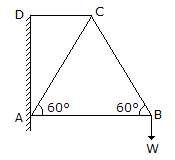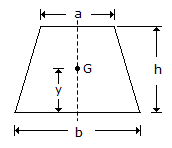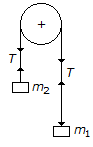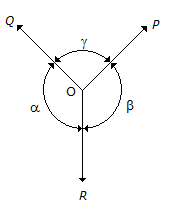Last updated: August 14, 2020

1. The unit of mass moment of inertia in S.I. units is kg-m2.
2. The skidding away of the vehicle on a level circular path can be avoided if the force of friction between the wheels and the ground is __________ the centrifugal force.
3. In a framed structure, as shown in the below figure, the force in the member AC is numerically equal to the force in member BC.4. The acceleration of a particle moving with simple harmonic motion, at any instant is given by (ω= angular velocity, y= displacement)
5. According to the law of moments, if a number of coplaner forces acting on a particle are in equilibrium, then
6. The centre of gravity of a trapezium with parallel sides a and b lies at a distance of y from the base b, as shown in the below figure. The value of y is7. Concurrent forces are those forces whose lines of action
8. Moment of inertia of a triangular section of base (b) and height (h) about an axis through its base, is
9. The velocity ratio of a differential pulley block with D and d as the diameters of larger and smaller pulley, is
10. The force which acts along the radius of a circle and directed towards the centre of the circle is known as centripetal force.
11. The centre of gravity of an isosceles triangle with base (p) and sides (q) from its base is
12. Tension in the cable supporting a lift is more when the lift is moving __________ with an acceleration.
13. The total momentum of a system of masses (i. e. moving bodies) in any one direction remains constant, unless acted upon by an external force in that direction. This statement is called
14. One newton is defined as the force while acting upon a mass of one kg, produces an acceleration of 1 m / s2 in the direction of which it acts.
15. If u1 and u2 are the velocities of two moving bodies in the same direction before impact and v1 and v2 are their velocities after impact, then coefficient of restitution is given by
16. A machine having an efficiency less than 50%, is known as
17. Two bodies of masses m1,and m2 are hung from the ends of a rope, passing over a friction-less pulley as shown in the figure below. The acceleration of the string will be18. The above figure shows the three coplaner forces P, Q and R acting at a point O. If these forces are in equilibrium, then
19. The angle of inclination with the vertical is __________ if the cyclist is running at a faster speed than that when he is running at a slower speed.
20. The unit of work in S.I. unit system is
Engineering Mechanics MCQ 5
You got {{userScore}} out of {{maxScore}} correct
{{title}}
{{image}}
{{content}}